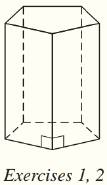Chapter 9.CT, Problem 2CTElementary Geometry For College St...

7th Edition
Alexander + 2 others
ISBN: 9781337614085

Solutions

Chapter
SectionElementary Geometry For College St...

7th Edition
Alexander + 2 others
ISBN: 9781337614085
Textbook Problem

For the regular pentagonal base, each edge measures 3.2 cm and the apothem measures 2 cm.a) Find the area of the base ( use  A = 1 2 a P ) . b) Find the total area of the regular pentagonal prism if its altitude measures 5 cm.c) Find the volume of the prism.To determine

a)

To find:

The area of the regular pentagonal base.

Explanation

Given:

For the regular pentagonal base, each edge measures 3.2 cm and the apothem measures 2 cm.

Formula used:

The area of the base is A=12aP where a is the length of the apothem and P is the perimeter of the base.

Calculation:

We have a=2 cm, s=3.2 cm.

Since there are 5 edges in the base, the perimeter of the base is P=53.2=16 cm

To determine

b)

To find:

The total area of the regular pentagonal prism.

To determine

c)

To find:

The volume of the prism.

Still sussing out bartleby?

Check out a sample textbook solution.

See a sample solution

The Solution to Your Study Problems

Bartleby provides explanations to thousands of textbook problems written by our experts, many with advanced degrees!

Get Started

Find more solutions based on key concepts